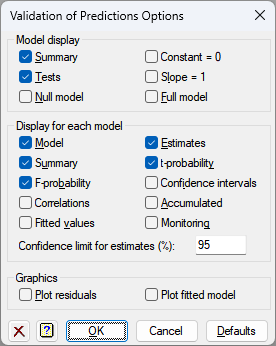1. Home
2. Validation of Predictions Options

# Validation of Predictions Options

Select the output to be generated initially in a Validation of Predictions analysis – the same information can also be displayed after the analysis, using the Further Output dialog.## Model Display

 Summary Summary of the four models fitted, showing the parameter estimates and their standard errors, the residual sum of squares, degrees of freedom and mean squares. Tests Tests to assess whether the constant differs from zero, and the slope differs from one. Null model Display the null model with the slope set to 1 and constant/intercept set to 0 Slope = 1 Display the model with the slope set to 1 and constant/intercept estimated from the data Zero constant Display the model with the constant/intercept set to 0 and slope estimated from the data Full model Display the full model with the slope and constant/intercept estimated from the data

The components displayed for each model selected above are controlled by the entries in the Display group box below.

## Display

 Model Details of the model that is fitted. Summary Summary analysis-of-variance. F-probability F probabilities for variance ratios. Correlations Correlations between the parameter estimates Fitted values Table containing the values of the response variate, the fitted values and residuals from the regression. Estimates Estimates of the parameters in the model t-probability t probabilities for the parameter estimates the fitted values, standardized residuals and leverages. Confidence intervals Confidence intervals for the parameter estimates. The confidence limit can specified as a percentage using the Confidence limit for estimates (%) field. Accumulated Analysis of variance table containing a line for each change in the fitted model.

## Graphics

Lets you generate default plots when you the analysis. Plot residuals will produce diagnostic plots of the residuals for each selected model. Plot fitted model to generates graphs of the fitted model for each selected model.